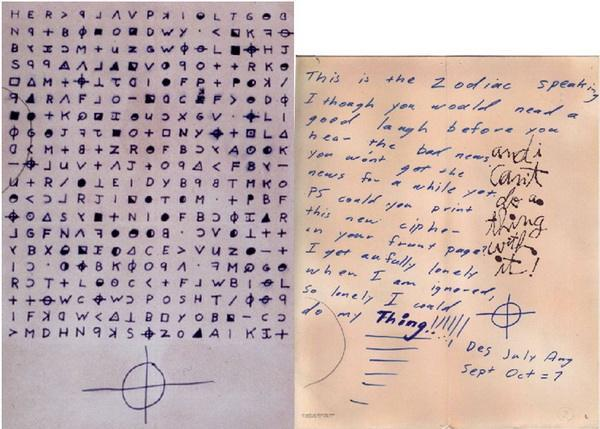## 计蒜客 – 重复的密文

1266 0 2020年3月10日

0 0 投票数

8
cabcabca

3

#include <bits/stdc++.h>

// 为了编程方便，next 数组下标从 1 开始，所以 string 也要下标从 1 开始，所以封装了一个 read
char* s = (char*)malloc(sizeof(char) * (length + 2));
for (int i = 1; i <= length; i++) {
char c;
scanf("%c", &c);
s[i] = c;
}
s[length + 1] = '\0';
return s;
}

// 以字符串 t 求 next 数组，n 为字符串长度
int* getNext(const char* t, int length) {
int* next = (int*)malloc(sizeof(int) * (length + 1));
next = 0;
for(int i = 2; i <= length; i++) {
int j = next[i - 1];
while(t[j + 1] != t[i] && j > 0) {
j = next[j];
}
if(t[j + 1] == t[i]) {
next[i] = j + 1;
} else {
next[i] = 0;
}
}
return next;
}

void print(const char * t, const int* next, int n) {
for (int i = 1; i <= n; i++) {
printf("%c%c", t[i], i == n ? '\n' : '\t');
}
for (int i = 1; i <= n; i++) {
printf("%d%c", next[i], i == n ? '\n' : '\t');
}
}

int main() {
int n;
scanf("%d\n", &n);
int* next = getNext(t, n);
//    print(t, next, n);
printf("%d\n", n - next[n]);
}
0 0 投票数（可选）如果您也有个人网站，不妨分享一下

0 评论

Warning: error_log(/home/wwwroot/www.jxtxzzw.com/wp-content/plugins/spider-analyser/#log/log-1105.txt): failed to open stream: No such file or directory in /home/wwwroot/www.jxtxzzw.com/wp-content/plugins/spider-analyser/spider.class.php on line 2900